# Allan

Allan keeps tropical fish. His aquarium is 4 feet long, 1 foot wide, and 2 feet tall. Each fish needs at least 0.5ft³ of water. What is the maximum number of fish that he can keep in the aquarium? Please show your solution. Please

Result

n =  16

#### Solution:Leave us a comment of example and its solution (i.e. if it is still somewhat unclear...):

Showing 3 comments:Math student
UnclearMath student
If (7+x) +7x=?Math student
Help solve## Next similar examples:

1. AquariumAquarium is rectangular box with square base containing 76 liters of water. Length of base edge is 42 cm. To what height the water level goes?
2. Jumps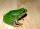At least how many jumps must do frog to overcome the distance of 7 meters? Jump of frog is 18 cm long.
3. StepsWalkway from the house to the school is 180 m long. How many more steps Michael makes than his father on the way to school, if the length of Michael's step is 60 cm and the length of his father's step is 90 cm.
4. RoundingThe following numbers round to the thousandth:
5. Round it0.728 round to units, tenths, hundredths.
6. Crates 2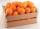One crate will hold 50 oranges. If Bob needs to ship 932 oranges, how many crates will he need?
7. Pool in litresPool has a width of 3.5 m length of 6 m and a height 1.60 meters. Calculate pool volume in liters.
8. Two cuboidsFind the volume of cuboidal box whose one edge is: a) 1.4m and b) 2.1dm
9. Truck of milk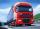How many hectoliters of ,,the box" milk fit in the truck, ake cargo size area is 2.8 m x 3 m x 17 m? A liter of milk in a box measuring 12 cm x 7 cm x 20 cm.
10. Pool coating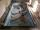How many pieces of tiles 25 cm × 15 cm need to coat the bottom and side walls of the pool with bottom dimensions 30 m × 5 m, if the pool can fit up to 271500 liters of water?
11. HectolitersHow many hectoliters of water fits into cuboid tank with dimensions of a = 3.5 m b = 2.5 m c = 1.4 m?
12. Water reservoirThe water tank has a cuboid with edges a= 1 m, b=2 m , c = 1 m. Calculate how many centimeters of water level falls, if we fill fifteen 12 liters cans.
13. Yogurt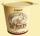The board is 35 yogurts. How many boards of yogurts must be ordered for 886 people in the canteen?
14. Fire tankHow deep is the fire tank with the dimensions of the bottom 7m and 12m, when filled with 420 m3 of water?
15. Caleb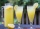Caleb is making lemonade for a party. He has 5 gallons of lemonade. He is putting 1/3 cubic inch of lemonade in a cup for each guest. How many guests are going to be at the party?
16. Jill's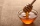Jill's mom stored some of the honey in a container that held 3/4 of a gallon. She used half of this amount to sweeten tea. How much honey, in cups was used in the tea. Express your answers in cups.
17. Tank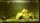The tank bottom has dimensions of 1.5 m and 3 2/6 m. The tank is 459.1 hl of water. How high is the water surface?